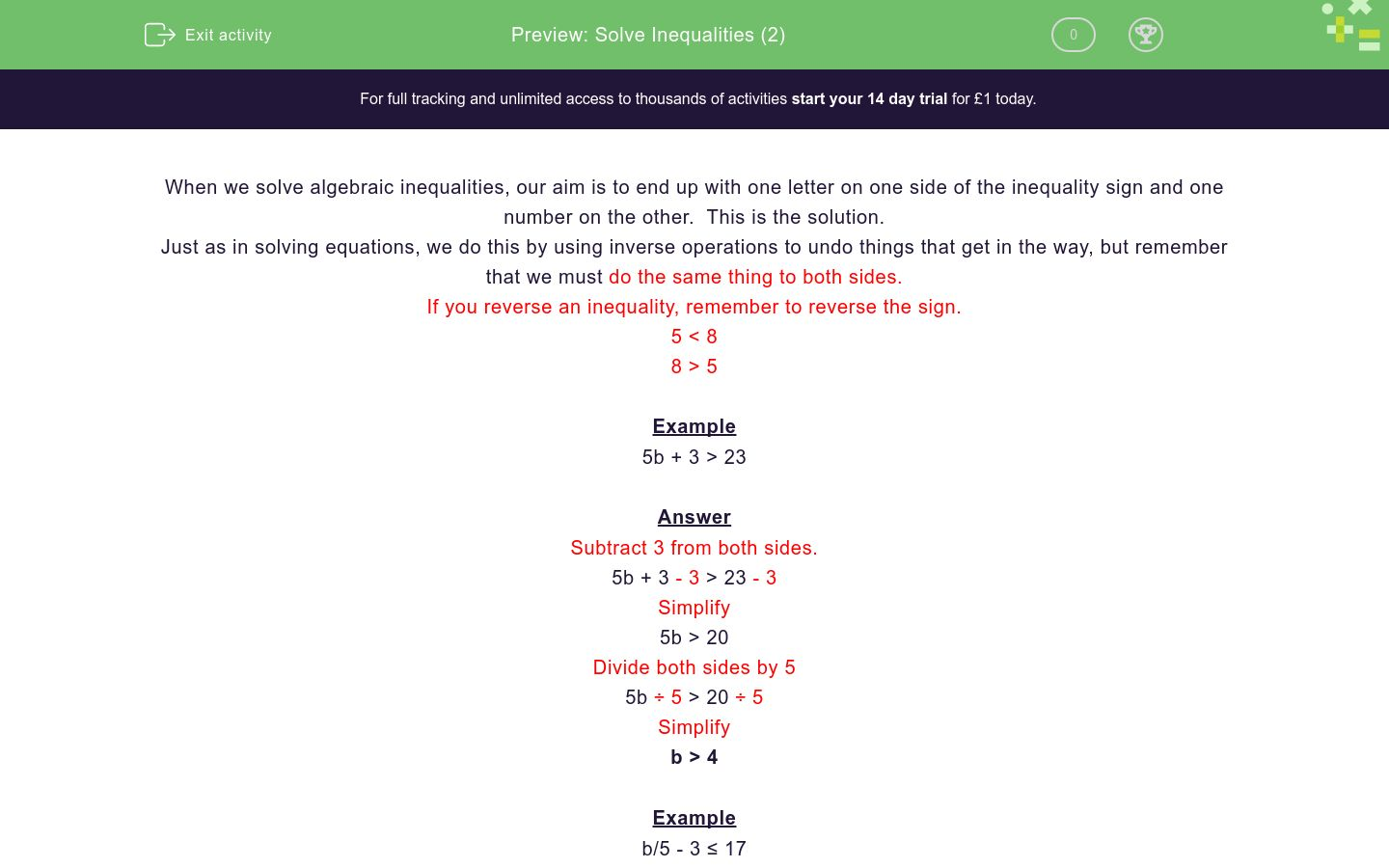# Solve Inequalities (2)

In this worksheet, students solve simple two-stage inequalities.Key stage:  KS 3

Curriculum topic:   Algebra

Curriculum subtopic:   Understand Expressions, Equations, Inequalities, Terms and Factors

Difficulty level:### QUESTION 1 of 10

When we solve algebraic inequalities, our aim is to end up with one letter on one side of the inequality sign and one number on the other.  This is the solution.

Just as in solving equations, we do this by using inverse operations to undo things that get in the way, but remember that we must do the same thing to both sides.

If you reverse an inequality, remember to reverse the sign.

5 < 8

8 > 5

Example

5b + 3 > 23

Subtract 3 from both sides.

5b + 3 - 3 > 23 - 3

Simplify

5b > 20

Divide both sides by 5

5b ÷ 5 > 20 ÷ 5

Simplify

b > 4

Example

b/5 - 3 ≤ 17

b/5 - 3 + 3 ≤ 17 + 3

Simplify

b/5 ≤ 20

Multiply both sides by 5

b ÷ 5 x 5 ≤ 20 x 5

Simplify

b ≤ 100

Example

Solve for a

5 - a ≥ 10

5 - a + a ≥ 10 + a

Simplify

5 ≥ 10 + a

Subtract 10 from both sides.

5 -10 ≥ 10 - 10 + a

Simplify

-5 ≥ a

REVERSE and BE CAREFUL to change the SIGN DIRECTION

a -5

---- OR ----